(The Long Way)

Celestial Navigation is a lost art, like cursive writing or speaking in sentences. Contrived reasons for learning celestial navigation—your GPS might fail—are not persuasive. So why not learn celestial navigation just to understand it, in other words for the beauty of the subject.Sometime around 1980 I asked my friend and mentor Captain Bob to present a short course on Celestial Navigation.  The course consisted of two or three lectures at my house, and one practice session with the sextant.  This article is compiled from notes that I took in Bob's course.

Our instructor explained his aim in the first lecture.  In a word the goal was
"understanding." Many explanations of celestial navigation aim to teach practical tools—ways of computing a line of position or fix while minimizing the computational burden—It is possible to acquire proficiency in such shortcut methods without understanding how they work.  To emphasize the difference between computational shortcuts and genuine understanding, I have subtitled this account of Captain Bob's course The Long Way.

The problem of celestial navigation may be summarized as follows: From your last known position you have sailed a course by dead reckoning (using a compass and log). Based on this information, that is, your last position, the compass course sailed, and the distance traveled (elapsed time multiplied by speed), you compute an assumed position (where you think you are).  The celestial navigation part, then, consists of combining your assumed position with one or more sextant sights to calculate a line of position (line containing your actual position).  If you have two or more sights at substantially different angles, or on different (heavenly) bodies, then it is possible to compute the current position without reference to an assumed position.

In school we learn that contrary to ancient beliefs, the earth is not the center of anything.  Copernicus, we are told, disproved that egotistical prejudice when he created the heliocentric theory, which put the sun at the center of the solar system. Well, forget Copernicus.  For navigational purposes, the earth is back at the center again.

The mathematical underpinnings of celestial navigation belong to a subject known as spherical trigonometry.  However, the part of trigonometry that we need for celestial navigation is very basic—only a small step from plane geometry, and easy to visualize with the help of diagrams.

Part 1 - Definitions and The Law of Cosines

Let's begin with some definitions.  Many of these terms are part of everyday language—we understand them already.

 Latitude and Longitude Positions on earth are specified using a pair of coordinate values called latitude and longitude.  Latitude refers to the angular distance north or south of the equator along a meridian (a great circle passing through the earth's poles).  Longitude refers to the angular distance east or west of the meridian that runs through Greenwich England.  Earth is for all practical purposes a sphere.  When specifying distances on a spherical surface, angle measure is often used.  From a navigational standpoint the primary unit of distance is the nautical mile, which by definition equals one minute of arc on earth's surface.  1 nautical mile is approximately 1.15 statute miles.The figure illustrates an angular distance on earth's surface.  C denotes the center of earth.  Angle ∠ACB is the angular distance from A to B, or the angle measure of the arc AB.

Any circle on earth's surface whose center is the same as earth's center is called a great circle.  Great circles describe the shortest distance between points on a spherical surface, just as straight lines do on a plane surface.  If the angle in the figure is 60
°, then the great circle distance between points A and B is 60 times 60 = 3600 nautical miles.  This great circle path from A to B is the shortest or most direct path from A to B on earth's surface.

Meridians of longitude are great circles.  However, the equator is the only great circle of latitude.  A corollary to this observation is that if one travels along a circle of latitude that is not the equator (say for example at latitude 30
° north), the distance traveled will be greater than along a great circle connecting the same origin and destination points.

 The celestial sphere Besides earth there is another sphere of importance in navigation. This one is imaginary, and is called the celestial sphere.  The celestial sphere is an enormous sphere whose center is earth.  The sun, moon, planets, and stars—all heavenly bodies—may be imagined to be located on the surface of this sphere.  (I told you to forget Copernicus!)  In relation to the celestial sphere, earth should be regarded as a single point at the center.  The celestial sphere is not meant as an accurate representation of the universe, but rather as a conceptual tool for navigational purposes.

Key reference points on earth have their couterparts on the celestial sphere. Projecting from earth's poles are a north and south celestial pole. Corresponding to earth's equator is the celestial equator.  Wherever you are on earth, the point in the sky directly above you is called the zenith.  The zenith is usually abbreviated with the letter Z and the point opposite the zenith (180
° from the zenith) is called the nadir, abbreviated Z' (pronounced Z-prime).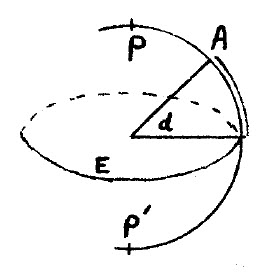Celestial meridians (projections of earth's meridian circles) are called hour circles.  Referring to the figure on the left, if A denotes a heavenly body (the sun for example), then the celestial latitude of A is called A's declination. Declination is the angular distance along the hour circle of A from the celestial equator. (In the figure P and P' are the celestial poles, and E the celestial equator.)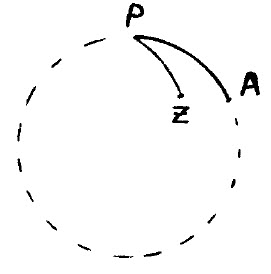Now refer to the figure on the right.  Again P is the celestial pole and Z the observor's zenith.  As in the previous figure, the heavenly body is denoted by A.  The angle ∠ZPA between the observor's celestial meredian and the hour circle of A is called the local hour angle of A (abbreviated LHA).  Similarly the angle between the Greenwich celestial meridian and the hour circle of A is called the Greenwich hour angle of A (abbreviated GHA).

Declination and Greenwich hour angle are the coordinates that specify the location of heavenly bodies as a function of date and time in the same way that latitude and longitude specify locations on earth.  Declinations and Greenwich hour angles for all navigational bodies are tabled in the Nautical Almanac, available for purchase in printed form as well as free on-line.

 Horizon The horizon is a great circle tangent to earth, with poles at the zenith and nadir.  The horizon and celestial meridian intersect at the north point and south point.  While the horizon and celestial equator intersect at the east point and west point. Vertical circle, altitude, and azimuth For any heavenly body A, the vertical circle of A is the great circle that passes through Z (the observor's zenith) and A.  The altitude of A is its angular distance from the horizon measured along the vertical circle of A. Finally, the azimuth of A is angle between the local meridian and the vertical circle of A, measured from 0 to 360 degrees eastward from the north point.  In other words,azimuth refers to the compass bearing from the observer to A.

The good news is that we are done with definitions, more-or-less.  The bad news is that we now have to do a little math, but nothing very difficult.  According to my 30+ year old notes, the mathematical starting point in Captain Bob's short course on celestial navigation was the law of cosines.  I am going to start one step before that with the Pythagorean Theorem.  Nearly everyone can recite the Pythagorean Theorem by rote, "In a right triangle, the square on the hypotenuse is equal to the sum of the squares on the other sides." Or, as a formula a2 = b2 + c2. But, if your life depended on it, could you prove that the theorem is true?  If in doubt, please stare at the drawing below for a few minutes.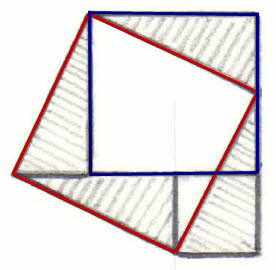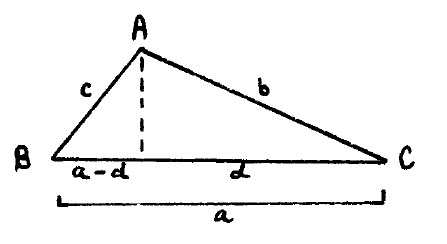The law of cosines may be viewed as a generalization of the Pythagorean theorem for oblique triangles.  Referring to the figure on the left, side a can be labeled in two parts as d and a - d.

Applying the Pythagorean theorem, b2 - d2 = c2 - (a - d)2 ... The shared side is the same!  Expanding the square on (a - d) and rearranging obtains a2 + b2 -2ad = c2 ...  Finally, making the substitution d/b = cos(C) produces the law of cosines:

c2 = a2 + b2 - 2ab ⋅ cos(C)

The law of cosines may be applied to solve for any of the three sides of an oblique triangle, provided the other two sides and the included angle are known.  This equation is all that is needed to derive the two main formulas of spherical trigonometry, which in turn are used to solve the problem of celestial navigation.

Part 2 - Applying Spherical Trigonometry

In this section the two formulas of spherical trigonometry that are needed to solve the celestial navigation problem will be derived.  Recall from Part 1 that a great circle is any circle on the surface of a sphere whose center is also the center of the sphere. The angle of intersection of two arcs of great circles is defined as the angle formed by the tangents to these arcs at their point of intersection.

The angle measure of an arc of a great circle is the measure of the angle subtended by the arc at the center of the sphere.  For spherical triangles, this measure is analogous to the length of a side in plane triangles.  Thus sides as well as angles of spherical triangles have angle measure. The actual length of the arc corresponding to the side of a spherical triangle may be easily computed when the radius and angle measure are known.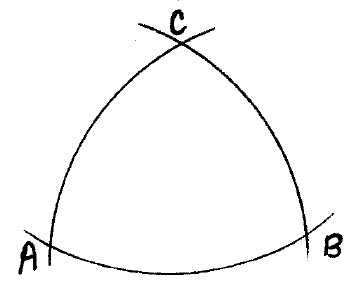Any three points on the surface of a sphere that are not on a great circle may be joined by arcs of great circles. The resulting figure is called a spherical triangle. This definition is analogous to the assertion that any three points on a plane that are not on a straight line may be connected by straight lines, making a plane triangle.  The figure on the left illustrates a spherical triangle with angles A, B, and C, and sides AB, BC, and CA.

From the standpoint of celestial navigation, two cases of solving the spherical triangle are important.  They are: 1. Given two sides and the included angle, and 2. Given three sides.  These two cases will be worked out in the context of the actual navigation problem.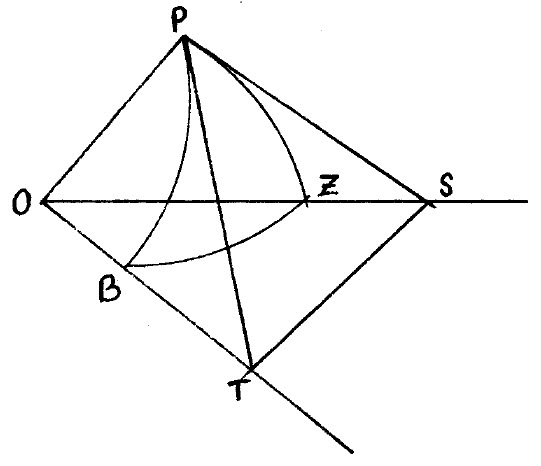In the figure above, O represents earth's center.  P is the celestial pole.  Z is the observer's zenith, that is the zenith of the observer's assumed position.  B is a celestial body, for example, the sun.  The line PT is tangent to the arc PB at P. Similarly PS is tangent to arc PZ at P.  Thus, angle P is the same as ∠TPS by the definition of spherical angles.  In the following paragraphs, the plane triangle formulas (Pythagorean Theorem and law of cosines) are applied to this figure to derive the spherical triangle formulas that are needed.

In this problem the following are known: The angle ∠PB (recalling that sides of spherical triangles are angles also), the angle ∠PZ, and the angle P.  What we want to know is the angle ∠ZB, which will give us the zenith distance of the body, and hence its altitude, since altitude (denoted Hc) is 90
° minus ZB.

PB is called the co-declination.  It is 90
° minus the declination of the body.  Recalling that declination is the body's distance (in angle measure) north or south of the celestial equator, and knowing that the equator is 90° from the pole, it follows immediately that PB is the co-declination.

Similarly, PZ is called the co-latitude.  It is 90
° minus the observer's assumed latitude. The explanation is analogous to that for co-declination.  Finally, angle P is the local hour angle of the body B, which may be computed by subtracting the (tabled) Greenwich Hour Angle (GHA) of the body from the assumed longitude.The drawing above summarizes these important terms from a different perspective.  In a sense this is the "everything" figure of celestial navigation.
BPG is the Greenwich Hour Angle (GHA) of B.  ZPG is the longitude of Z.  ZPB is the computed Local Hour Angle (LHA) of B.  EB is the declination of B, PB the co-declination.  ZF is the observer's latitude and PZ the co-latitude.  BH is the computed altitude of the body (Hc).  Note that Hc is 90° minus ZB, the zenith distance of the body. (The zenith is 90° above the horizon.)

Now back to the previous spherical triangles figure (the one with O at the center) ... Examination of this figure confirms the following correspondences: Arc ZB  of the celestial triangle PBZ is equal to plane angle
BOZ.  Similarly, PZ = ∠ZOP, and PB = ∠POB.  These three equalities establish  a correspondence between plane angles and arcs of the celestial triangle PBZ.

Applying the law of cosines to the plane triangle OTS obtains:

(1)  OT2 + OS2 - 2OTOS  cos(ZB) = ST2

Similarly applying the law of cosines to the plane triangle PTS obtains:

(2)  PT2 + PS2 - 2PTPS  cos(P) = ST2

Now subtract equation (2) from equation (1) and slightly rearrange terms to get:

(3)  (OT2 - PT2) + (OS2 - PS2) +  2PTPS  cos(P) = 2OTOS  cos(ZB)

We are almost home on the first of the two spherical triangle formulas.  Observe that angles
OPT and OPS are right angles.  If this is not obvious, think about the 3-dimensional meaning of the drawing, where the point O represents earth and point P the celestial pole.  Since OPT is a right angle, by the Pythagorean theorem, OT2 - PT2 is OP2.  And since OPS is a right angle, OS2 - PS2 is also OP2.  Therefore we can substitute OP2 for the each of the differences and re-write expresssion (3) as:

(4)  2OP22PTPS  cos(P) = 2OTOS  cos(ZB)

Now divide expression (4) by 2
OTOS to obtain:

(5)  (OP/OT)⋅(OP/OS) + (PT/OT)⋅(PS/OS)⋅cos(P) = cos(ZB)

Expression (5) might be clearer if it were exhibited with the numerator on one line, then a horizontal line for divide and the denominator underneath.  However, I don't know how to do that in HTML at this point.  So as written, terms that will be substituted in the next step are grouped together.  Note that OP/OT is cos(PB).  Again, if this is not obvious, think about the 3-dimensional meaning of the drawing—OT is the hypotenuse of the right triangle OTP (the right angle is at P).  Similarly, OP/OS = cos(PZ), PT/OT = sin(PB) and PS/OS = sin(PZ).  This takes us to:

(6)  cos(ZB) = cos(PB)cos(PZ) + sin(PB)sin(PZ)cos(P)

Equation (6) gives the zenith altitude of the body ZB, and hence the computed altitude 90° - ZB = Hc.  It is the main formula for solving any spherical triangle, given two sides and the included angle.

As explained in the preceding paragraph, the first of the two spherical triangle formulas gives the computed altitiude of the body Hc.  The next formula will yield the azimuth of the body.  The angle
PZB or Z for short is the bearing toward the body from Z.  To obtain an expression for this angle, first invert expression (6), solving for cos(P).  Again, it would be preferable to display this equation using a horizontal line for divide, but using what I know it is:

(7)  cos(P) = (cos(ZB) - cos(PB)cos(PZ)) / (sin(PB)sin(PZ))

Although expressed in terms of the polar angle P, formula (7) is the general solution of a spherical triangle whenever the three sides are known.  Thus we are free to transpose sides, PB for ZB, PZ for PB, and ZB for PZ, obtaining:

(8)  cos(P) = (cos(PB) - cos(PZ)cos(ZB)) / (sin(PZ)sin(ZB))

Equation (8) gives the azimuth of the body from the assumed position.  To review, PB is the co-declination, PZ is the co-latitude, and ZB is the zenith distance of the body.  When using equation (6), the first of the spherical triangle formulas, the zenith distance is unknown, while the local hour angle (LHA) of the body is computed from assumed longitude and the (tabled) Greenwich Hour Angle (GHA).  On the other hand, when using equation (8), the second of the spherical triangle formulas, one computes the zenith distance by subtracting the sextant height (the observed altitude of the body, including corrections) from 90
°.  The declination and assumed latitude remain known, and the formula is used to compute the zenith angle or azimuth of the body.

Equations (6) and (8) are the two main formulas of celesital navigation.

Part 3 - Line of Position and Beyond

The celestial navigation problem was stated in the fourth paragraph of the introduction (here).  To compute a line of position (LOP) it is necessary to reconcile a computed estimate of the body's altitude Hc with an observed measure of its altitude
Ho (a sextant sight).  The algebraic sign of the difference between Hc and Ho indicates whether your true position is closer to the body or further away from the body than your assumed position.  You will also know that you are somewhere on a line (actually a large circle) whose azimuth (bearing toward the body) agrees with the bearing computed from observational data.

Thus a LOP (solution to the celestial navigation problem) specifies two values: 1) distance in nautical miles "toward" or "away" from the body, 2) an azimuth angle or bearing toward the body from your position.  These values are easily transcribed to produce a line on the navigational chart on which you have plotted your dead reckoning course and position.

Most navigational sights are taken on the sun (or that was the case when navigational sights were taken!).  However, the moon, planets, and bright stars (navigational stars) may also be used.  The reason why stars are not used much is that the horizon cannot be seen in darkness.  Therefore the height of stars above the horizon can only be measured at dawn and dusk, when both the star and horizon are visible.

The Nautical Almanac tables the GHA of the sun, moon, planets, and Aries, for every second of the year, as well as various correction factors for which limb of the sun or moon was sighted, height of the eye, parallax, and so forth.

Aries is the celestial reference point for fixed stars.  By definition, Aries (also called the vernal equinox) is the point of intersection of the celestial equator and the ecliptic. The Nautical Almanac tables the sidereal hour angle (SHA) of all navigational stars.  SHA is the angle between the meridian of the star and the meridian of Aries, measured westward. Astronomers use Right Ascension (RA), which is the eastward angle and is expressed in units of time rather than degrees.  Since the SHA of fixed stars does not vary appreciably in the course of a year, the tabled GHA for Aries suffices to compute the GHA, and hence the LHA of any navigational star.

Part 4 - Summary

The overall theory of celestial navigation was described in Parts 1 and 3 and the main formulas
were derived in Part 2.  To become proficient at practical celestial navigation also requires taking practice sights with a sextant.  It is most helpful to have a computer or programmable calculator to carry out the tedious computational steps (especially if using The Long Way).  Celestial navigation software is commonplace now.  However, in the past, when it was truly needed, there was no such thing!  If doing calculations by hand (using tables but not a computer program) it is easy to make errors.  Fortunately such errors almost always produce absurd results, positions that could not possibly be correct—thousands of miles away.  One of the commonest types of error is to accidentally invert a sign, substituting plus for minus, or the reverse.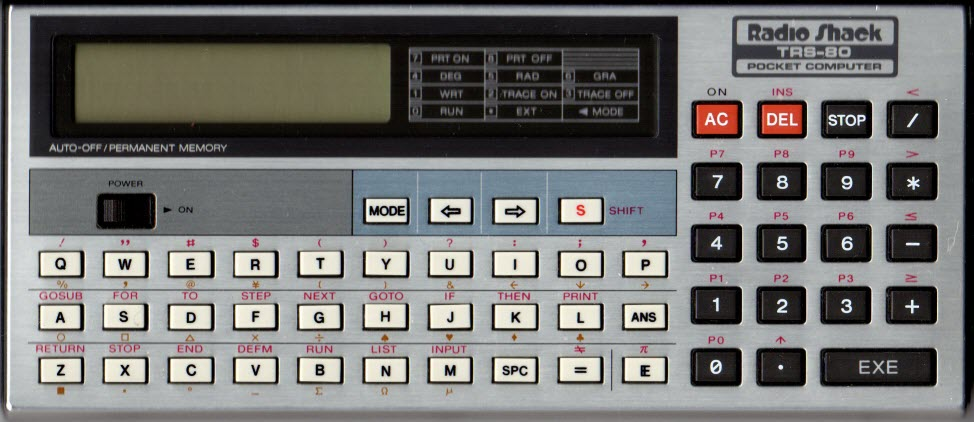By the late 1980's I had acquired a programmable pocket computer, the Radio Shack TRS-80 model PC-4 pictured above.  This little computer had a built-in BASIC language interpreter, and 1 or 2 KB of memory.  I programmed the Line of Position problem, and later, dead reckoning and fix (intersection of two circles of position). Eventually I got all the navigational problems to fit in memory, with a single user interface (if it could be called that).  A facsimile scan of the original Line of Position program from my old PC-4 notebook together with a contemporary computer BASIC translation of the program and a worked example are reproduced here.

 Errors in this recounting of course notes are mine.
 Later, as is well known, Kepler showed that the sun is at one focus of an ellipse.
 The unshaded part of the drawing represents overlapping of square c (the hypotenuse) with squares a and b (the other sides).  Matching correspondingly shaded parts shows that square c is equal to the sum of squares a and b.
 I am fortunate to live near the ocean, and therefore have a real horizon for practice sights.  To practice taking sextant sights without a real horizon it is possible to measure the angle between a body and its reflection in a pool of water or a mirror.  Then halve the angle measure to obtain an estimate of the horizon angle.